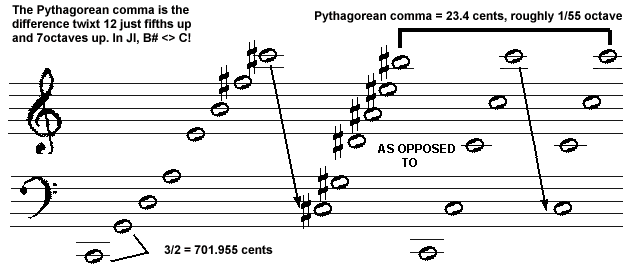## Definitions of tuning terms © 1998 by Joseph L. Monzo All definitions by Joe Monzo unless otherwise cited

Pythagorean Comma

Commas are small musical intervals between a note formed by one cycle of just intervals and the same note formed by another cycle of different just intervals. These commas prove important in constructing musical scales, and in notating musical chords and musical pitches.

The Pythagorean comma is the difference between 12 just perfect fifths up and 7 octaves up:[from Brian McLaren]

McLaren's description can be shown in both vector notation and in regular fractional math thus:

```    2   3

[-12  12]       (3/2)12 = 312/212 = 531441/4096      531441/4096    12 "5ths"
- [  7   0]      ÷ (2/1)7  = 128                     *      1/128      7 "8ves"
-----------  =  ---------                        =  ---------------
[-19  12]                                           531441/524288   Pythagorean comma

```

...........................

The ratio 531441/ 524288, in JustMusic prime-factor notation designated as 312, with an interval size of approximately 0.23 Semitones [= 23.460+ cents].

It is the difference between the Pythagorean or 3-Limit "tritone" or "augmented 4th" of approximately 6.12 Semitones [= 729/512 = 36 = 611.730+ cents] and the Pythagorean or 3-Limit "diminished 5th" of approximately 5.88 Semitones [= 1024/729 = 3-6 = ~588.2699948 cents], shown below in both vector notation and in regular fractional math:

```for C = n0 = 1/1 :

2  3

[ -9  6]       (9/8)3          = 93/83           =  729/512       729/512      tritone: F:B
- [ 10 -6]     ÷ (223-1)/(2-835) = (4/3)/(243/256) = 1024/729    *  729/1024     "diminished 5th": B:F
----------  =  ---------                                      =  -------------
[-19 12]                                                       531441/524288   Pythagorean comma

```

It was first described c. 300 BC by pseudo-Euclid in Divisions of the Canon.

Below are some other interval measurements for the Pythagorean comma:

```
~0.234600104  (~1/4) Semitone
~1.407600623  (~1 2/5) 72edo-moria
~5.865002596  (~5 6/7) savarts
~14.27150632  (~14 1/4) 730edo Woolhouse-units
~19.55000865  (~19 5/9) milli8ves
~20.72300917  (~20 5/7) Türk cents
~23.46001038  (~23 1/2) cents
~588.5139105  (~588 1/2) jots
720 tuning units
~960.9220254  (~961 71/77) cawapus
~3843.688101  (~3843 2/3) midipus
```

[from Joe Monzo, JustMusic: A New Harmony]

updated:

2002.09.28 -- added table of interval measurements
2002.09.22 -- added vector notation for tritone explanation
2002.09.07 -- added vector notation for McLaren's explanation
1999.12.16

 I welcome feedback about this webpage: corrections, improvements, good links. Let me know if you don't understand something.return to the Microtonal Dictionary indexreturn to my home pagereturn to the Sonic Arts home page